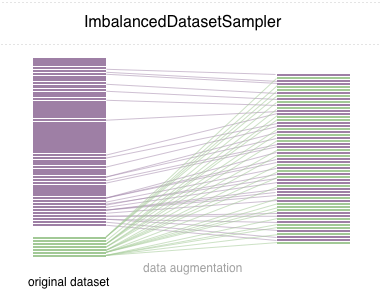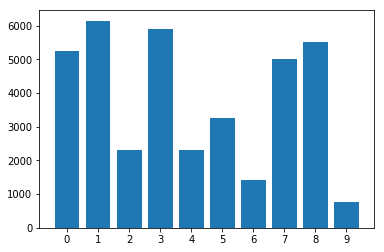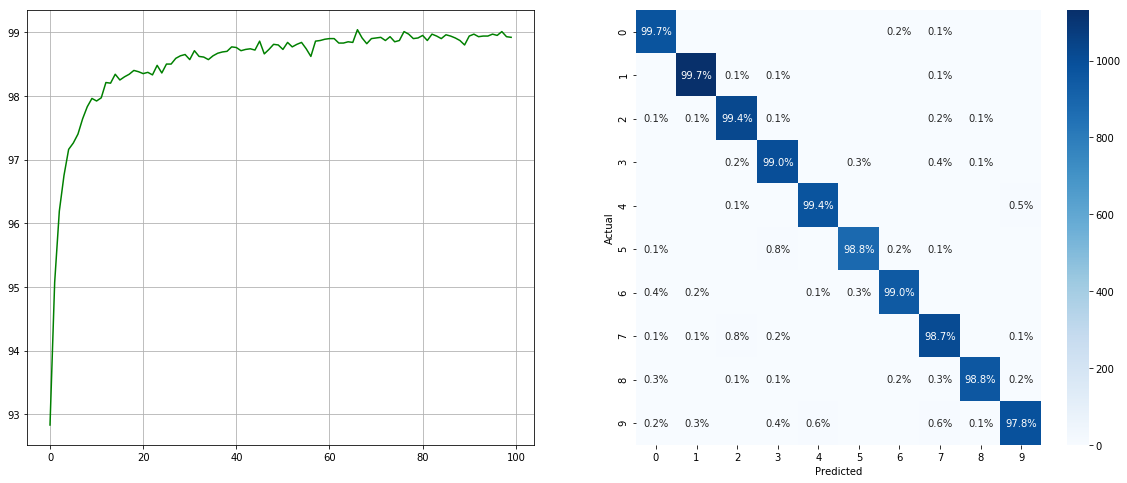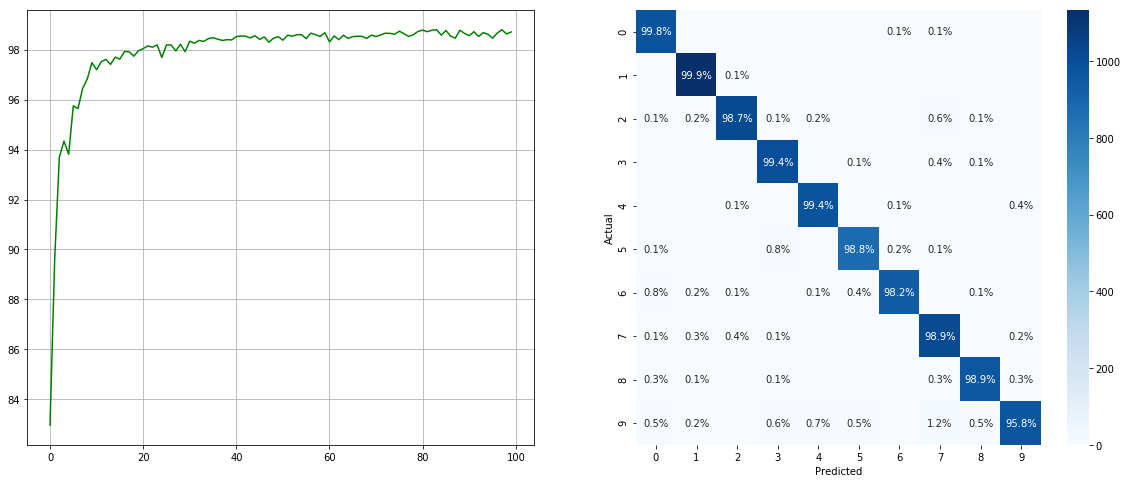## Imbalanced Dataset Sampler

A (PyTorch) imbalanced dataset sampler for oversampling low frequent classes and undersampling high frequent ones.

In many machine learning applications, we often come across datasets where some types of data may be seen more than other types. Take identification of rare diseases for example, there are probably more normal samples than disease ones. In these cases, we need to make sure that the trained model is not biased towards the class that has more data. As an example, consider a dataset where there are 5 disease images and 20 normal images. If the model predicts all images to be normal, its accuracy is 80%, and F1-score of such a model is 0.88. Therefore, the model has high tendency to be biased toward the ‘normal’ class.

To solve this problem, a widely adopted technique is called resampling. It consists of removing samples from the majority class (under-sampling) and / or adding more examples from the minority class (over-sampling). Despite the advantage of balancing classes, these techniques also have their weaknesses (there is no free lunch). The simplest implementation of over-sampling is to duplicate random records from the minority class, which can cause overfitting. In under-sampling, the simplest technique involves removing random records from the majority class, which can cause loss of information.## Usage

Simply pass an `ImbalancedDatasetSampler` for the parameter `sampler` when creating a `DataLoader`.
For example:

``````from sampler import ImbalancedDatasetSampler

train_dataset,
sampler=ImbalancedDatasetSampler(train_dataset),
batch_size=args.batch_size,
**kwargs
)
``````

Then in each epoch, the loader will sample the entire dataset and weigh your samples inversely to your class appearing probability.

## Example: Imbalanced MNIST Dataset

Distribution of classes in the imbalanced dataset:With Imbalanced Dataset Sampler:(left: test acc in each epoch; right: confusion matrix)

Without Imbalanced Dataset Sampler:(left: test acc in each epoch; right: confusion matrix)

Note that there are significant improvements for minor classes such as `2` `6` `9`, while the accuracy of the other classes is preserved.